Refer to our Texas Go Math Grade 1 Answer Key Pdf to score good marks in the exams. Test yourself by practicing the problems from Texas Go Math Grade 1 Unit 4 Assessment Answer Key.

Vocabulary

Write the words that describe the shape. (p.572)
equal parts
unequal parts

Question 1.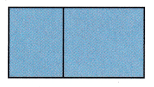They are unequal parts.

Explanation:
First part size of the given figure is smaller than the second part size .
=> They are unequal parts.

Question 2.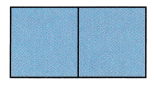They are equal parts.

Explanation:
First part size of the given figure is equal to the second part size .
=> They are equal parts.

Concepts and Skills

Question 3.
Circle the shapes that can combine to make the new shape. (TEKS 1.6.F)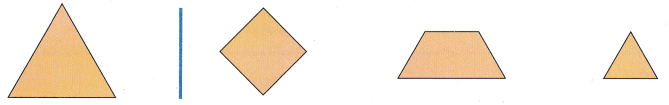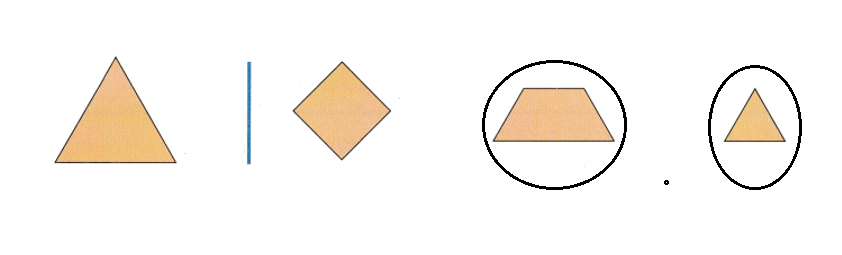Explanation:
Trapezium combined with triangle can form a new shape quadrilateral.

Question 4.
Draw a line under the objects that have both flat and curved surfaces. (TEKS 1.6.E)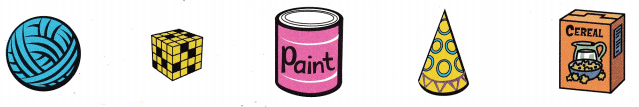Explanation:
A curve is a shape or a line which is smoothly drawn in a plane having a bent or turns in it.
For example, a circle is an example of curved-shape.
A closed two-dimensional, or flat, figure is called a plane shape.

Question 5.
Draw lines to show 2 equal parts. (TEKS 1.6.G,1.6.H)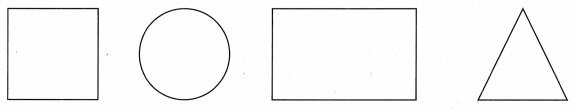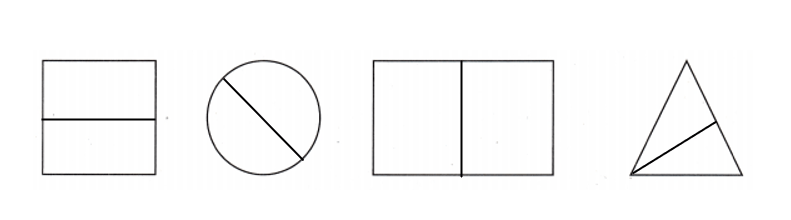Explanation:
Dividing the figure into two equal length is called dividing it into two equal parts.

Question 6.
Which shading shows a fourth of a rectangle? (TEKS 1.6.G, 1.6.H)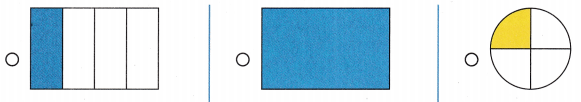Explanation:
When one (1) whole is divided into 4 equal parts each part is called one quarter (or one fourth).

Question 7.
Mike uses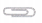to measure his pencil. About how long is the pencil? (TEKS 1.7.B, 1.7.D)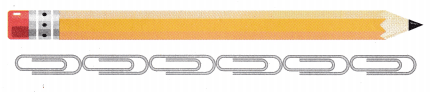(A) about 4(B) about 6(C) about 2About sixis the length of pencil.
(B) about 6.

Explanation:
Number ofMike uses to measure his pencil = 6.

Question 8.
How many vertices does a hexagon have? (TEKS 1.6.D)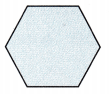(A) 4
(B) 6
(C) 5
Hexagon has six vertices.
(B) 6.

Explanation:
Vertices is the plural of the word vertex, which is the point at which two or more lines/edges meet. Edges are straight lines that connect one vertex to another. Faces are the flat surfaces of shapes.
Hexagon has six vertices.

Question 9.
Which flat surface does a cube have? (TEKS 1.6.E)Explanation:
There are six flat surfaces that are the skin of a cube. Each flat surface is called a face.
Square flat surface a cube has.

Question 10.
A box is the same length as this string. Use string to find which object will fit in the box.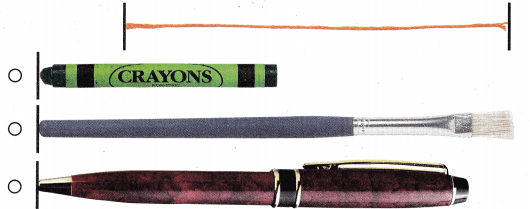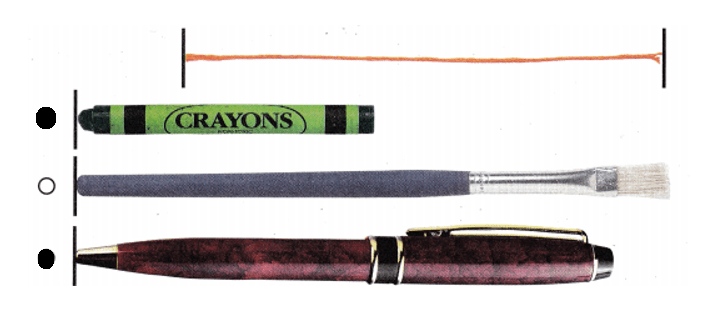Explanation:
The string length is equal to the pen and the crayon is smaller than it also.
So, both can fit in the box.

Question 11.
What is the time? (TEKS 1.7.E)(A) 10 o’clock
(B) 4 o’clock
(C) 12 o’clock
Time shown in the clock: 4:00.
(B) 4 o’clock

Explanation:
Hours hand is showing: 4.
Minutes hand is showing: 12.Question 12.
Which shows halves? (TEKS 1.6.G, 1.6.H)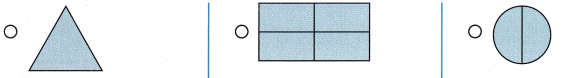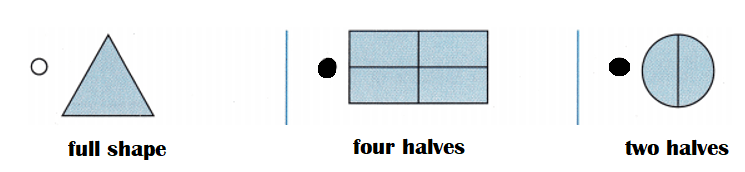Explanation:
Triangle is full shape no halves.
Rectangle is divided into four halves.
Circle is divided into two halves.

Question 13.
What time is it? (TEKS 1.7.E)(A) 6 o’clock
(B) half past 12
(C) 12 o’clock
Time shown in the clock = 12:30 or half past 12.
(B) half past 12.

Explanation:
Hours hand is showing: 12 and 1 between.
Minutes hand is showing: 6.

Question 14.
Which solid has 9 edges? (TEKS 1.6.E)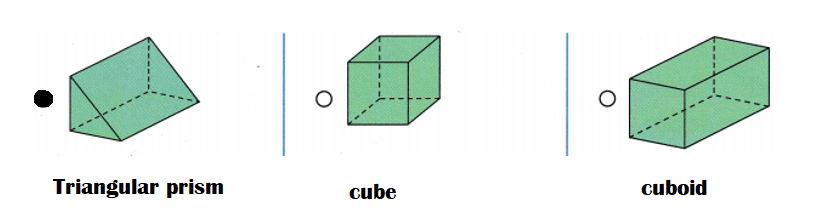Explanation:
A Triangular Prism has 9 edges.
A cube has 12 edges.
A cuboid has 12 edges.

Question 15.
Why does the straw use fewer units to measure? (TEKS 1.7.C)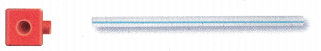(A) the straw is shorter so it uses more units
(B) the straw is longer so it uses fewer units
(C) the straw is the same length so it uses the same number of units
Straw is longer so it uses fewer units to measure than the cube.
(B) the straw is longer so it uses fewer units

Explanation:
The straw is longer so it uses fewer units to measure than the cube.

Question 16.
Which solid matches the riddle? (TEKS 1.6.E)
I am a block.
I have 6 faces that are squares.Explanation:
A cylinder has two flat ends in the shape of circles. Cylinder has 2 faces.

A cube has six equal, square-shaped sides. Cubes also have eight vertices (corners) and twelve edges, all the same length. cube has 6 faces.
I am a block.
I have 6 faces that are squares.

A triangular prism is a three-sided prism; it is a polyhedron made of a triangular base, a translated copy, and 3 faces joining corresponding sides. A right triangular prism has rectangular sides, otherwise it is oblique. A triangular prism has 5 faces.

Question 17.
Use the following clues to draw a shape

• The shape has only straight sides.
• The shape has more than 3 sides.
• The shape has fewer than 5 corners.
• The shape can be cut into fourths.

Draw to show the shape. Draw lines to show how the shape can be cut. Write how many equal shares there are Justify. Explain why your answer is reasonable. (TEKS 1.6.A, 1.6.D, 1.6.G)What shapes are the equal shares?
Square has equal sides.

Explanation:
The shape has only straight sides.
The shape has more than 3 sides.
The shape has fewer than 5 corners.
The shape can be cut into fourths.
According to this points, it can be a Square or Rectangle.How many corners does one equal share have?